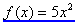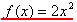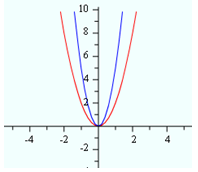Effects of "a"

When the a-value is greater than one, the parabola becomes narrower. When the a-value is less than one, the parabola becomes wider. When the a-value is positive, the parabola will open upward; when it is negative the parabola will open downward.
Example
The graph below represents the functionin blue andin red. Since both a -values are positive, the parabolas open up. Since 5 > 2, the blue parabola will be narrower than the red parabola.Listen